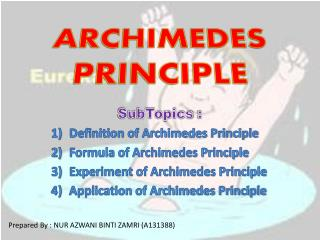DownloadDownload PresentationARCHIMEDES PRINCIPLE

# ARCHIMEDES PRINCIPLE

Download Presentation## ARCHIMEDES PRINCIPLE

- - - - - - - - - - - - - - - - - - - - - - - - - - - E N D - - - - - - - - - - - - - - - - - - - - - - - - - - -
##### Presentation Transcript

1. ARCHIMEDES PRINCIPLE SubTopics : Definition of Archimedes Principle Formula of Archimedes Principle Experiment of Archimedes Principle Application of Archimedes Principle Prepared By : NUR AZWANI BINTI ZAMRI (A131388)

2. DEFINITION OF ARCHIMEDES PRINCIPLE An object is immersed in a fluid is buoyed up by a force equal to the weight of the fluid displaced by the object.

3. FORMULA OF ARCHIMEDES PRINCIPLE FB = wA –wF Where ; FB is again the buoyant force on the object, wA is the normal weight of the object measured in air (wA = m g), and wF is the weight taken while the object is immersed in a fluid of density rF.

4. SIMPLE EXPERIMENT OF PRINCIPLE ARCHIMEDES

5. CALCULATION From the equation : FB = wA –wF According to experiment ; wA = 640g , Wf = 410g(by referring to water displacement,230g ) FB = wA –wF = 640g – 410g = 230g

6. STATE OF BOUYANCY Buoyancy is always an upward force created by a fluid. Positive • If an object floats, it means the object displaces an amount of water that weighs more than the object does. In other words, the object is less dense than the water. Negative • If an object displaces an amount of water that weighs less than the object does, it sinks. Because the object is denser than the water, the water does not provide sufficient buoyancy to make it hover or float. Neutral • If an object is neutral, it hovers. This means the object displaces an amount of water that weighs the same as it does.

7. WATCH THIS VIDEO!

8. APPLICATION OF PRINCIPLE ARCHIMEDES 1) SUBMARINE A submarine has a large ballast tank, which is used to control its position and depth from  the surface of the sea. A submarine submerges by letting water into the ballast tank so that its weight becomes greater than the buoyant force (and vice versa). It floats by reducing water in the ballast tank.-thus its weight is less than the buoyant force.

9. 2.HOT AIR BALLON - The atmosphere is filled with air that exerts buoyant force on any object. - A hot air balloon rises and floats due to the buoyant force (when the surrounding air is greater than its weight). It descends when the balloon weight is more than the buoyant force. It becomes stationary when the weight = buoyant force. - The weight of the Hot-air balloon can be controlled by varying the quantity of hot air in the balloon.

10. 3. SHIP - A ship floats on the surface of the sea because the volume of water displaced by the ship is enough to have a weight equal to the weight of the ship.- A ship is constructed in a way so that the shape is hollow, to make the overall density of the ship lesser than the sea water. Therefore, the buoyant force acting on the ship is large enough to support its weight.

11. CONCLUSION By Archimedes’ principle,Upthrust on body = Weight of fluid displacedTherefore,Weight of floating body = Weight of fluid displacedThis result, sometimes called the “principle of floatation”, is a special case of Archimedes’ principle and can be stated: A floating body displaces its own weight of fluid. When a body is completely or partially immersed in a fluid it experiences an upthrust, or an apparent loss in weight, which is equal to the weight of fluid displaced.

12. REFERENCES